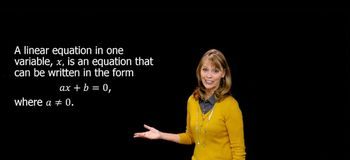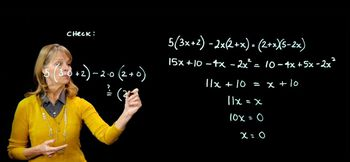Start typing, then use the up and down arrows to select an option from the list.
5:28 minutes
Problem 89
Textbook Question

# The equations in Exercises 79–90 combine the types of equations we have discussed in this section. Solve each equation. Then state whether the equation is an identity, a conditional equation, or an inconsistent equation. 4x/(x + 3) - 12/(x - 3) = (4x^2 + 36)/(x^2 - 9)Verified Solution
This video solution was recommended by our tutors as helpful for the problem above.
33views